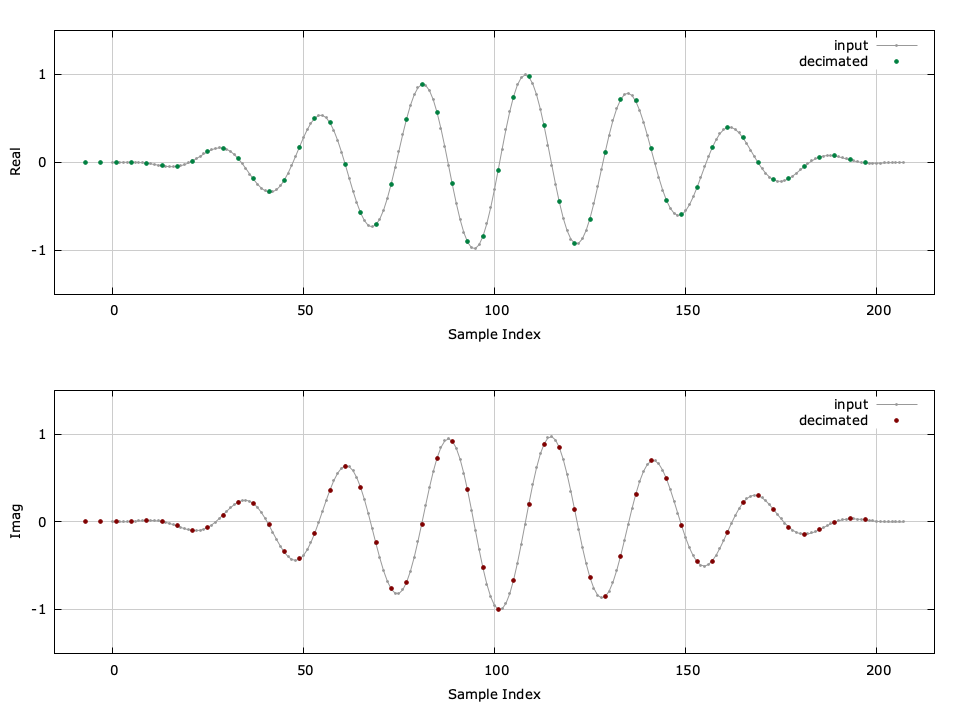# infinite impulse response decimator (iirdecim)

The iirdecim object family implements a basic interpolator with an integer output-to-input resampling ratio $$M$$ . It is similar to the firdecim family but with an infinite impulse response (recursive) filter performing the alias rejection. In essense, the iirdecim family is essentially just a iirfilt object which operates on a block of samples at a time. An example of the iirdecimator can be seen in [ref:fig-filter-iirdecim-crcf] .Figure [fig-filter-iirdecim-crcf]. iirdecim_crcf (iirdecimator) example with $$M=4$$ , compensating for filter delay.

Listed below is the full interface to the iirdecim family of objects. While each method is listed for iirdecim_crcf , the same functionality applies to iirdecim_rrrf and iirdecim_cccf .

• iirdecim_crcf_create(M,*b,nb,*a,na) creates a iirdecim object with a decimation factor $$M$$ using the filter coefficients defined by $$\vec{a}$$ and $$\vec{b}$$ .
• iirdecim_crcf_create_default(M,order) creates a iirdecim object from a prototype filter with a decimation factor $$M$$ and a filter order $$order$$ . The default filter is a Butterworth design.
• iirdecim_crcf_create_prototype(M,ftype,btype,format,order,fc,f0,Ap,As) creates a iirdecim object from a prototype with a decimation factor $$M$$ . See iirdes for details on the filter design parameters.
• iirdecim_crcf_destroy(q) destroys a iirdecim object, freeing all internally-allocated memory.
• iirdecim_crcf_print(q) prints the parameters of a iirdecim object to the standard output.
• iirdecim_crcf_reset(q) clears the internal buffer of a iirdecim object.
• iirdecim_crcf_execute(q,*x,*y) computes the output decimation of the input sequence $$\vec{x}$$ (which is $$M$$ samples in size) and stores the single output sample result in $$y$$ .
• iirdecim_crcf_execute_block(q,*x,n,*y) computes the output decimation of the input sequence $$\vec{x}$$ (which is $$nM$$ samples in size) and stores the resulting $$n$$ samples in $$y$$ .
• iirdecim_crcf_groupdelay(q,fc) computes the filter group delay at frequency $$f_c$$ .

An example of the iirdecim interface is listed below.

#include <liquid/liquid.h>

int main() {
// options
unsigned int M = 4;     // decimation factor
unsigned int n = 21;    // filter length

// design filter and create decimator object
iirdecim_crcf q = iirdecim_crcf_create_default(M,n);

// generate input signal and decimate
float complex x[M];         // input samples
float complex y;            // output sample

// run decimator (repeat as necessary)
{
iirdecim_crcf_execute(q, x, &y);
}

// destroy decimator object
iirdecim_crcf_destroy(q);
}


A more detailed example is given in examples/iirdecim_crcf_example.c in the main liquid project directory.